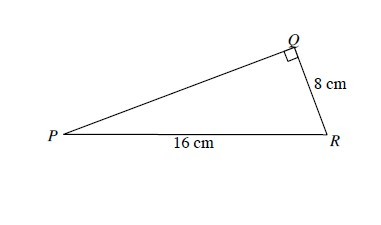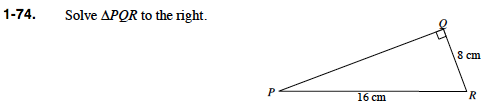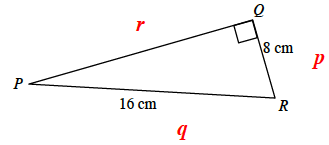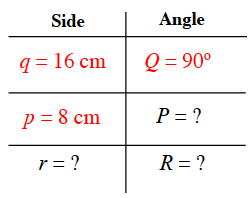### Home > PC > Chapter 1 > Lesson 1.2.1 > Problem1-74

1-74.

Solve ΔPQR below. Homework Help ✎First label the triangle with lower case letters for the sides.What does it mean to solve a triangle?

Make a chart. Fill in with known information. Then find the unknown values.

Find the lengths of all 3 sides and the 3 angle measurements.How could you find Angle P?

Once you have two angles in the right triangle, how do you find the 3rd angle?

In a right triangle, what famous theorem helps in finding a third side if two sides are already known?

Use the Sine function or the Law of Sines.

180° (the sum of the first two angles)

Pythagorean Theorem
a² + b² = c²